# The Curse of Dimensionality

## Followed by an intro to dimensionality reduction!

How do machines ‘see’? Or, in general, how can computers reduce an input of complex, high-dimensional data into a more manageable number of features?

Extend your open hand in front of a nearby light-source, so that it casts a shadow against the nearest surface. Rotate your hand and study how its shadow changes. Note that from some angles it casts a narrow, thin shadow. Yet from other angles, the shadow looks much more recognizably like the shape of a hand.

See if you can find the angle which best projects your hand. Preserve as much of the information about its shape as possible.

Behind all the linear algebra and computational methods, this is what dimensionality reduction seeks to do with high-dimensional data. Through rotation you can find the optimal angle which represents your 3-D hand as a 2-D shadow.

There are statistical techniques which can find the best representation of data in a lower-dimensional space than that in which it was originally provided.

In this article, we will see why this is an often necessary procedure, via a tour of mind-bending geometry and combinatorics. Then, we will examine the code behind a range of useful dimensionality reduction algorithms, step-by-step.

My aim is to make these often difficult concepts more accessible to the general reader — anyone with an interest in how data science and machine learning techniques are fast changing the world as we know it.

Semi-supervised machine learning is a hot topic in the field of data science, and for good reason. Combining the latest theoretical advances with today’s powerful hardware is a recipe for exciting breakthroughs and science fiction evoking headlines.

We may attribute some of its appeal to how it approximates our own human experience of learning about the world around us.

The high-level idea is straightforward: given information about a set of labelled “training” data, how can we generalize and make accurate inferences about a set of previously “unseen” data?

Machine learning algorithms are designed to implement this idea. They use a range of different assumptions and input data types. These may be simplistic like K-means clustering. Or complex like Latent Dirichlet Allocation.

Behind all semi-supervised algorithms though are two key assumptions: continuity and embedding. These relate to the nature of the feature space in which the data are described. Below is a visual representation of data points in a 3-D feature space.

Higher dimensional feature spaces can be thought of as scatter graphs with more axes than we can draw or visualize. The math remains more or less the same!

Continuity is the idea that similar data points such as those which are near to each other in ‘feature space’ are more likely to share the same label. Did you notice in the scatter graph above that nearby points are similarly colored? This assumption is the basis for a set of machine learning algorithms called clustering algorithms.

Embedding is the assumption that although the data may be described in a high-dimensional feature space such as a ‘scatter-graph-with-too-many-axes-to-draw’, the underlying structure of the data is likely much lower-dimensional.

For example, in the scatter graph above we have shown the data in 3-D feature space. But the points fall more or less along a 2-D plane.

Embedding allows us to effectively simplify our data by looking for its underlying structure.

Apart from having both the coolest and scariest sounding name in all data science, the phenomena collectively known as the Curse of Dimensionality also pose real challenges to practitioners in the field.

Although somewhat on the melodramatic side, the title reflects an unavoidable reality of working with high-dimensional data sets. This includes those where each point of data is described by many measurements or ‘features’.

The general theme is simple — the more dimensions you work with, the less effective standard computational and statistical techniques become. This has repercussions that need some serious workarounds when machines are dealing with Big Data. Before we dive into some of these solutions, let’s discuss the challenges raised by high-dimensional data in the first place.

Working with data becomes more demanding as the number of dimensions increases. Like many challenges in data science, this boils down to combinatorics.

With n = 1, there are only 5 boxes to search. With n = 2, there are now 25 boxes; and with n = 3, there are 125 boxes to search. As n gets bigger, it becomes difficult to sample all the boxes. This makes the treasure harder to find — especially as many of the boxes are likely to be empty!

In general, with n dimensions each allowing for m states, we will have m^n possible combinations. Try plugging in a few different values and you will be convinced that this presents a workload-versus-sampling challenge to machines tasked with repeatedly sampling different combinations of variables.

With high-dimensional data, we simply cannot comprehensively sample all the possible combinations, leaving vast regions of feature space in the dark.

## Dimensional Redundancy

We may not even need to subject our machines to such demanding work. Having many dimensions is no guarantee that every dimension is especially useful . A lot of the time, we may be measuring the same underlying pattern in several different ways.

For instance, we could look at data about professional football or soccer players. We may describe each player in six dimensions.

This could be in terms of:

• number of goals scored
• number of of shots attempted
• number of chances created
• number of tackles won

There are six dimensions. Yet you might see that we are actually only describing two underlying qualities — offensive and defensive ability — from a number of angles.

This is an example of the embedding assumption we discussed earlier. High dimensional data often has a much lower-dimensional underlying structure.

In this case, we’d expect to see strong correlations between some of our dimensions. Goals scored and shots attempted will unlikely be independent of one another. Much of the information in each dimension is already contained in some of the others.

Often high-dimensional data will show such behavior. Many of the dimensions are, in some sense, redundant.

Highly correlated dimensions can harmfully impact other statistical techniques which rely upon assumptions of independence. This could lead to much-dreaded problems such as over-fitting.

Many high-dimensional data sets are actually the results of lower-dimensional generative processes. The classic example is the human voice. It can produce very high-dimensional data from the movement of only a small number of vocal chords.

High-dimensionality can mask the generative processes. These are often what we’re interested in learning more about.

Not only does high-dimensionality pose computational challenges, it often does so without bringing much new information to the show.

And there’s more! Here’s where things start getting bizarre.

## Geometric Insanity

Another problem arising from high-dimensional data concerns the effectiveness of different distance metrics, and the statistical techniques which depend upon them.

This is a tricky concept to grasp, because we’re so used to thinking in everyday terms of three spatial dimensions. This can be a bit of a hindrance for us humans.

Geometry starts getting weird in high-dimensional space. Not only hard-to-visualize weird, but more “WTF-is-that?!” weird.

Let’s begin with an example in a more familiar number of dimensions. Say you’re mailing a disc with a diameter of 10cm to a friend who likes discs. You could fit it snugly into a square envelope with sides of 10cm, leaving only the corners unused. What percentage of space in the envelope remains unused?

Well, the envelope has an area of 100cm² inside it, and the disc takes up 78.5398… cm² (recall the area of a circle equals πr²). In other words, the disc takes up ~78.5% of the space available. Less than a quarter remains empty in the four corners.

Now say you’re packaging up a ball which also has a diameter of 10cm, this time into a cube shaped box with sides of 10cm. The box has a total volume of 10³ = 1000cm³, while the ball has a volume of 523.5988… cm³ (the volume of a 3-D sphere can be calculated using 4/3 * πr³). This represents almost 52.4% of the total volume available. In other words, almost half of the box’s volume is empty space in the eight corners.

See these examples below: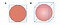See how there is more empty space left in the cube than in the square?

The volume of a sphere in 3-D is smaller in example B than that of a circle in the 2-D example B. The center of a cube is smaller than the center of a square with the same length side. Does this pattern continue in more than three dimensions? Or when we’re dealing with hyper-spheres and hyper-cubes? Where do we even begin?

Let us think about what a sphere actually is, mathematically speaking. We can define an n-dimensional sphere as the surface formed by rotating a radius of fixed length r about a central point in (n+1)-dimensional space.

In 2-D, this traces out the edge of circle which is a 1-D line. In 3-D this traces out the 2-D surface of an everyday sphere. In 4-D+, which we cannot easily visualize, this process draws out a hyper-sphere.

It’s harder to picture this concept in higher dimensions, but the pattern which we saw earlier continues . The relative volume of the sphere diminishes.

The generalized formula for the volume of a hyper-sphere with radius r in n dimensions is shown below:

Γ is the Gamma function, described here. Technically, we should be calling volume in > 3 dimensions hyper-content.

The volume of a hyper-cube with sides of length 2r in n dimensions is simply (2r)^n. If we extend our sphere-packaging example into higher dimensions, we find the percentage of overall space filled can be found by the general formula:

We’ve taken the first formula, multiplied by 1 / (2r)^n and then cancelled where r^n appears on both sides of the fraction.

Look at how we have n/2 and n as exponents on the numerator (“top”) and denominator (“bottom”) of that fraction respectively. We can see that as n increases, the denominator will grow quicker than the numerator. This means the fraction gets smaller and smaller. That’s not to mention the fact the denominator also contains a Gamma function featuring n.

The Gamma function is like the factorial function… you know, the one where
(n! = 2 x 3 x … x n). The Gamma function also tends to grow really quickly. In fact, Γ(n) = (n-1)!.

This means that as the number of dimensions increases, the denominator grows much faster than the numerator. So the volume of the hyper-sphere decreases towards zero.

In case you don’t much feel like calculating Gamma functions and hyper-volumes in high dimensional space, I’ve made a quick graph:

The volume of the hyper-sphere (relative to the space in which it lives) rapidly plummets towards zero. This has serious repercussions in the world of Big Data.

…Why?

Recall our 2-D and 3-D examples. The empty space corresponded to the “corners” or “outlying regions” of the overall space.

For the 2-D case, our square had 4 corners which were 21.5% of the total space.

In the 3-D case, our cube now had 8 corners which accounted for 47.6% of the total space.

As we move into higher dimensions, we will find even more corners. This will make an ever increasing percentage of the total space available.

Now imagine we have data spread across some multidimensional space. The higher the dimensionality, the higher the total proportion of our data will be “flung out” in the corners, and the more similar the distances will be between the minimum and maximum distances between points.

In higher dimensions our data are more sparse and more similarly spaced apart. This makes most distance functions less effective.

# Escaping the Curse!

There are a number of techniques which can project our high-dimensional data into a lower dimensional space. Recall the analogy of a 3-D object placed in front of a light source projects a 2-D shadow against a wall.

By reducing the dimensionality of our data, we make three gains:

• less dimensional redundancy
• more effective distance metrics

No wonder dimensionality reduction is so crucial in advanced machine learning applications such as computer vision, NLP and predictive modelling.

We’ll walk through five methods which are commonly applied to high-dimensional data sets. We’ll be restricting ourselves to feature extraction methods. They try to identify new features underlying the original data.

Feature selection methods choose which of the original features are worth keeping. We’ll leave those for a different article!

This is a long read with plenty of worked examples. So open your favorite code editor, put the kettle on, and let’s get started!

# Multidimensional Scaling (MDS)

## Visual Summary

MDS refers to family of techniques used to reduce dimensionality. They project the original data in a lower-dimensional space, while preserving the distances between the points as much as possible. This is usually achieved by minimizing a loss-function (often called stress or strain) via an iterative algorithm.

Stress is a function which measures how much of the original distance between points has been lost. If our projection does a good job at retaining the original distances, the returned value will be low.

## Worked Example

If you have R installed, whack it open in your IDE of choice. Otherwise, if you want to follow along anyway, check this R-fiddle.

We’ll be looking at CMDS (Classical MDS) in this example. It will give an identical output to PCA (Principal Components Analysis), which we’ll discuss later.

We’ll be making use of two of R’s strengths in this example:

• working with matrix multiplication
• the existence of inbuilt data sets

`M <- as.matrix(UScitiesD)`

We want to begin with a distance matrix where each element represents the Euclidean distance (think Pythagoras’ Theorem) between our observations. The `UScitiesD` and `eurodist` data sets in R are straight-line and road distance matrices between a selection of U.S. and European cities.

With non-distance input data, we would need a preliminary step to calculate the distance matrix first.

`M <- as.matrix(dist(raw_data))`

With MDS, we seek to find a low-dimensional projection of the data that best preserves the distances between the points. In Classical MDS, we aim to minimize a loss-function called `Strain`.

Strain is a function that works out how much a given low-dimensional projection distorts the original distances between the points.

With MDS, iterative approaches (for example, via gradient descent) are usually used to edge our way towards an optimal solution. But with CMDS, there’s an algebraic way of getting there.

Time to bring in some linear algebra. If this stuff is new to you, don’t worry — you’ll pick things up with a little practice. A good starting point is to see matrices as blocks of numbers that we can manipulate all at once, and work from there.

Matrices follow certain rules for operations. Addition and multiplication can be broken down or decomposed into eigenvalues and corresponding eigenvectors.

Eigen-what now?

A simple way of thinking about all this eigen-stuff is in terms of transformations. Transformations can change both the direction and length of vectors upon which they act.

Shown below, matrix A describes a transformation, which is applied to two vectors by multiplying A x v. The blue vector’s direction of 1 unit across and 3 units up remains unchanged. Only it’s length changes, here it doubles. This makes the blue vector an eigenvector of A with an eigenvalue of 2.

The orange vector does change direction when multiplied by A, so it cannot be an eigenvector of A.

Back to CMDS — our first move is to define a centering matrix that lets us double center our input data. In R, we can implement this as below:

`n <- nrow(M)C <- diag(n) - (1/n) * matrix(rep(1, n^2), nrow = n)`

We then use R’s support for matrix multiplication `%*%` to apply the centering matrix to our original data to form a new matrix, B.

`B <- -(1/2) * C %*% M %*% C`

Nice! Now we can begin building our 2-D projection matrix. To do this, we define two more matrices using the eigenvectors associated with the two largest eigenvalues of matrix B.

Like so:

`E <- eigen(B)\$vectors[,1:2]L <- diag(2) * eigen(B)\$values[1:2]`

Let’s calculate our 2-D output matrix X, and plot the data according to the new co-ordinates.

`X <- E %*% L^(1/2)plot(-X, pch=4)text(-X, labels = rownames(M), cex = 0.5)`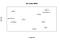Feel free to overlay a map of the U.S.and check everything lines up as it should!

How does that look? Pretty good, right? We have recovered the underlying 2-D layout of the cities from our original input distance matrix. Of course, this technique lets us use distance matrices calculated from even higher-dimensional data sets!

# Principal Components Analysis (PCA)

## Visual Summary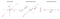Rotate the axes to describe as much of the variation in the data as possible. We retain most of the variation in the data using only one of our new axes.

In a large data set with many dimensions, some of the dimensions may well be correlated and essentially describe the same underlying information. We can use linear algebra to project our data into a lower-dimensional space, while retaining as much of the underlying information as possible.

The visual summary above provides a low-dimensional explanation. In the plot on the left, our data are described by two axes, x and y.

In the middle plot, we rotate the axes through the data in the direction that captures as much variation as possible. The new PC1 axis describes much more of the variation than axis PC2. In fact, we could ignore PC2 and still keep a large percentage of the variation in the data.

## Worked Example

Let’s use a small scale example to illustrate the core idea. In an R session or in this snippet at R-fiddle), let’s load one of the in-built data sets.

`data <- as.matrix(mtcars)head(data)dim(data)`

Here we have 32 observations of different cars across 11 dimensions. They include features and measurements such as mpg, cylinders, horsepower….

But how many of those 11 dimensions do we actually need? Are some of them correlated?

Let’s calculate the correlation between the number of cylinders and horsepower. Without any prior knowledge, what might we expect to find?

`cor(mtcars\$cyl, mtcars\$hp)`

That’s an interesting result . At +0.83, we find the correlation coefficient is pretty high. This suggests that number of cylinders and horsepower are both describing the same underlying feature. Are more of our dimensions doing something similar?

Let’s correlate all pairs of our dimensions and build a correlation matrix. Because life’s too short.

`cor(data)`

Each cell contains the correlation coefficient between the dimensions at each row and column. The diagonal always equals 1.

Correlation coefficients near +1 show strong positive correlation. Coefficients near -1 show strong negative correlation. We can see some values close to -1 and +1 in our correlation matrix. This shows we have some correlated dimensions in our data set.

This is cool, but we still have the same number of dimensions we started with. Let’s throw out a few!

To do this, we can get out the linear algebra again. One of the strong points of the R language is that it is good at linear algebra, and we’re gonna make use of that in our code. Our first step is to take our correlation matrix and find its eigenvalues.

`e <- eigen(cor(data))`

Let’s inspect the eigenvalues:

`e\$valuesbarplot(e\$values/sum(e\$values),main="Proportion Variance explained")`

We see 11 values which decrease pretty dramatically on the bar plot! We see that the eigenvector associated with the largest eigenvalue explains about 60% of the variation in our data. The eigenvector associated with the second largest eigenvalue explains about 24% of the variation in our original data. That’s already 84% of the variation in the data, explained by two dimensions!

OK, let’s say we want to keep 90% of the variation in our original data set. How many dimensions do we need to keep to achieve this?

`cumulative <- cumsum(e\$values/sum(e\$values))print(cumulative)i <- which(cumulative >= 0.9)print(i)`

We calculate the cumulative sum of our eigenvalues’ relative proportion of the total variance. We see that the eigenvectors associated with the 4 largest eigenvalues can describe 92.3% of the original variation in our data.

This is useful! We can retain >90% of the original structure using only 4 dimensions. Let’s project the original data set onto a 4-D space. To do this, we need to create a matrix of weights, which we’ll call W.

`W <- e\$vectors[1:ncol(data),1:i]`

W is an 11 x 4 matrix. Remember, 11 is the number of dimensions in our original data, and 4 is the number we want to have for our transformed data. Each column in W is given by the eigenvectors corresponding to the four largest eigenvalues we saw earlier.

To get our transformed data, we multiply the original data set by the weights matrix W. In R, we perform matrix multiplication with the %*% operator.

`tD <- data %*% Whead(tD)`

We can view our transformed data set . Now each car is described in terms of 4 principal components instead of the original 11 dimensions. To get a better understanding of what these principal components are actually describing, we can correlate them against the original 11 dimensions.

`cor(data, tD[,1:i])`

We see that component 1 is negatively correlated with cylinders, horsepower and displacement. It is also positively correlated with mpg and possessing a straight (as opposed to V-shaped) engine. This suggests that component 1 is a measure of engine type.

Cars with large, powerful engines will have a negative score for component 1. Smaller engines and more-fuel efficient cars will have a positive score. Recall that this component describes approximately 60% of the variation in the original data.

Likewise, we can interpret the remaining components in this manner. It can become trickier (if not impossible) to do so as we proceed. Each subsequent component describes a smaller and smaller proportion of the overall variation in the data. Nothing beats a little domain-specific expertise!

There are several aspects in which PCA can vary to the method described here. You can read an entire book on the subject.

# Linear Discriminant Analysis (LDA)

## Visual Summary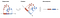With LDA, we want to find axes which best separate different classes of data

On the original axis, the red and blue classes overlap. Through rotation, we can find a new axis which better separates the classes. We may choose to use this axis to project our data into a lower-dimensional space.

PCA seeks axes that best describe the variation within the data. Linear Discriminant Analysis (LDA) seeks axes that best discriminate between two or more classes within the data.

This is achieved by calculating two measures

• within-class variance
• between-class variance.

The objective is to optimize the ratio between them. There is minimal variance within each class and maximal variance between the classes. We can do this with algebraic methods.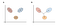Finding axes which minimizes the ratio between these measures is the goal of Linear Discriminant Analysis.

As shown above, A is the within-class scatter. B is the between-class scatter.

## How Does It Work?

Let’s generate a simple data set for this example (for the R-fiddle, click here).

`require(dplyr)languages <- data.frame(HTML = c(22,20,15, 5, 5, 5, 0, 2, 0),                  JavaScript = c(20,25,25,20,20,15, 5, 5, 0),                        Java = c(15, 5, 0,15,30,30,10,10,15),                      Python = c( 5, 0, 2, 5,10, 5,40,35,30),job = c("Web","Web","Web","App","App","App","Data","Data","Data"))View(languages)`

We have a fictional data set describing nine developers in terms of the number of hours they spend working in each of four languages:

• HTML
• JavaScript
• Java
• Python

Each developer is classed in one of three job roles:

• web developer
• app developer
• and data scientist
`cor(select(languages, -job))`

We use the `select()` function from the `dplyr` package to drop the class labels from the data set. This allows us to inspect the correlations between the different languages.

Unsurprisingly, we see some patterns. There is a strong, positive correlation between HTML and JavaScript. This indicates developers who use one of these languages have a tendency to also use the other.

We suspect that there is some lower-dimensional structure beneath this 4-D data set. Remember, four languages = four dimensions.

Let’s use LDA to project our data into a lower-dimensional space that best separates the three classes of job roles.

First, we need to build within-class scatter matrices for each class. Let’s use `dplyr`’s `filter()` and `select()` methods to break down our data by job role.

`Web <- as.data.frame(scale(filter(languages, job == "Web") %>% select(., -job),T))App <- as.data.frame(scale(filter(languages, job == "App") %>% select(., -job),T))Data <- as.data.frame(scale(filter(languages, job == "Data") %>% select(., -job),T))`

So now we have three new data sets, one for each job role. For each of these, we can find a covariance matrix. This is closely related to the correlation matrix. It also describes the trends between how languages are used together.

We find the within-class scatter matrix by summing the each of the three covariance matrices. This gives us a matrix describing the scatter within each class.

`within <- cov(Web) + cov(App) + cov(Data)`

Now we want to find the between-class scatter matrix which describes the scatter between the classes. To do this, we must first find the center of each class, by calculating the average features of each. This lets us form a `data.frame` where each column describes the average developer for each class.

`means <- t(data.frame(  mean_Web <- sapply(Web, mean),  mean_App <- sapply(App, mean),  mean_Data <- sapply(Data, mean)))`

To get our between-class scatter matrix, we find the covariance of this matrix.:

`between <- cov(means)`

Now we have two matrices:

• our within-class scatter matrix
• the between-class scatter matrix

We want to find new axes for our data which minimizes the ratio between within-class scatter and between-class scatter.

We do this by finding the eigenvectors of the matrix formed by:We multiply the inverse of the within-class scatter matrix SW by the between-class scatter matrix SB
`e <- eigen(solve(within) %*% between)barplot(e\$values/sum(e\$values),main='Variance explained')W <- e\$vectors[,1:2]`

By plotting the eigenvalues, we can see that the first two eigenvectors will explain >95% of the variation in the data.

Let’s transform the original data set and plot the data in its new, lower-dimensional space.

`LDA <- scale(select(languages, -job), T) %*% Wplot(LDA, pch="", main='Linear Discriminant Analysis')text(LDA[,1],LDA[,2],cex=0.75,languages\$job, col=unlist(lapply(c(2,3,4),rep, 3)))`

There you go! See how the new axes do an amazing job separating the different classes? This reduces the dimensionality of the data and could also prove useful for classification purposes.

To being interpreting the new axes, we can correlate them against the original data:

`cor(select(languages,-job),LDA)`

This reveals how Axis 1 is negatively correlated with JavaScript and HTML, and positively correlated with Python. This axis separates the Data Scientists from the Web and App developers.

Axis 2 is correlated with HTML and Java in opposite directions. This separates the Web developers from the App developers. It would be an interesting insight, if the data weren’t fictional…!

We have assumed the three classes are all equal in size, which simplifies things a bit. LDA can be applied to 2 or more classes, and can be used as a classification method as well.

Get the full picture and coverage of LDA’s use in classification.

# Non-linear Dimensionality Reduction

The techniques covered so far are pretty good in many use-cases, but they make a key assumption: that we are working in the context of linear geometry. Sometimes, this is an assumption we need to drop.

Non-linear dimensionality reduction (NLDR) opens up a fascinating world of advanced mathematics and mind-bending possibilities in applications such as computer vision and autonomy.

There are many NLDR methods available. We’ll take a look at a couple of techniques relating to manifold learning. These will approximate the underlying structure of high-dimensional data. Manifolds are one of the many mathematical concepts that might sound impenetrable but which are actually seen everyday.

Take this map of the world: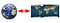Strictly speaking, the surface of a sphere requires one map of each hemisphere to be properly mapped…

We’re all fine with the idea of representing the surface of a sphere on a flat sheet of paper. Recall from before that a sphere is defined as a 2-D surface traced a fixed distance around a point in 3-D space. The earth’s surface is a 2-D manifold embedded, or wrapped around, in a 3-D space.

With high-dimensional data, we can use the concept of manifolds to reduce the number of dimensions we need to describe the data.

Think back to the surface of the earth. Earth exists in a 3-D space, so we should describe the location, such as a city, in three dimensions. However, we have no trouble using only two dimensions of latitude and longitude instead.

Manifolds can be more complex and higher-dimensional than the earth example here. Isomap and Laplacian Eigenmapping are two closely related methods used to apply this thinking to high-dimensional data.

# Isomap

## Visual Summary

We can see our original data as a U-shaped underlying structure. The straight-line distance, as shown by the black arrow, between A and B won’t reflect the fact they lie at opposite ends, as shown by the red line.

We can build a nearest-neighbors graph to find the shortest path between the points. This lets us build a distance matrix that can be used as an input for MDS to find a lower-dimensional representation of the original data that preserves the non-linear structure.

We can approximate distances on the manifold using techniques in graph theory. We can do this by building a graph or network by connecting each of our original data points to a set of neighboring points.

By using a shortest-paths algorithm, we can find the geodesic distance between each point. We can use this to form a distance matrix which can be an input for a linear dimensionality reduction method.

## Worked Example

We’re going to implement a simple Isomap algorithm using an artificially generated data set. We’ll keep things in low dimensions, to help visualize what is going on. Here’s the code.

Let’s start by generating some data:

`x <- y <- c(); a <- b <- 1for(i in 1:1000){    theta <- 0.01 * i    x <- append(x,(a+b*theta)*(cos(theta)+runif(1,-1,1))    y <- append(y,(a+b*theta)*(sin(theta)+runif(1,-1,1))}color <- rainbow(1200)[1:1000]spiral <- data.frame(x,y,color)plot(y~x, pch=20, col=color)`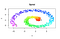Points A and B are at opposite ends of the spiral. The straight-line distance between them doesn’t reflect this.

Nice! That’s an interesting shape, with a clear, non-linear structure. Our data could be seen as scattered along a 1-D line, running between red and violet, coiled up (or embedded) in a 2-D space. Under the assumption of linearity, distance metrics and other statistical techniques won’t take this into account.

How can we unravel the data to find its underlying 1-D structure?

`pc <- prcomp(spiral[,1:2])plot(data.frame(pc\$x[,1],1),col=as.character(spiral\$color))`

PCA won’t help us, as it is a linear dimensionality reduction technique. See how it has collapsed all the points onto an axis running through the spiral? Instead of revealing the underlying red-violet spectrum of points, we only see the blue points scattered along the whole axis.

Let’s try implementing an Isomap algorithm. We begin by building a graph from our data points, by connecting each to its n-nearest neighboring points. n is a hyper-parameter that we need to set in advance of running the algorithm. For now, let’s use n = 5.

We can represent the n-nearest neighbors graph as an adjacency matrix A.

The element at the intersection of each row and column can be either 1 or 0 depending on whether the corresponding points are connected.

Let’s build this with the code below:

`n <- 5distance <- as.matrix(dist(spiral[,1:2]))A <- matrix(0,ncol=ncol(distance),nrow=nrow(distance))for(i in 1:nrow(A)){   neighbours <- as.integer(names(sort(distance[i,])[2:n+1]))   A[i,neighbours] <- 1}`

Now we have our n-nearest neighbors graph, we can start working with the data in a non-linear way. For example, we can begin to approximate the distances between points on the spiral by finding their geodesic distance — calculating the length of the shortest path between them.

Dijkstra’s algorithm is a famous algorithm which can be used to find the shortest path between any two points in a connected graph. We could implement our own version here but to remain on-topic, I will use the `distances()` function from R’s igraph library.

`install.packages('igraph'); require(igraph)graph <- graph_from_adjacency_matrix(A)geo <- distances(graph, algorithm = 'dijkstra')`

This gives us a distance matrix. Each element represents the shortest number of edges or links required to get from one point to another.

Here’s an idea… why not use MDS to find some co-ordinates for the points represented in this distance matrix? It worked earlier for the cities data.

We could wrap our earlier MDS example in a function and apply our own, homemade version. However, you’ll be pleased to know that R provides an in-built MDS function we can use as well. Let’s scale to one dimension.

`md <- data.frame('scaled'=cmdscale(geo,1), 'color'=spiral\$color)plot(data.frame(md\$scaled,1), col=as.character(md\$color), pch=20)`

We’ve reduced from 2-D to 1-D, without ignoring the underlying manifold structure. For advanced, non-linear machine learning purposes, this is a big deal. Often enough, high-dimensional data arises as a result of a lower-dimensional generative process. Our spiral example illustrates this.

The original spiral was plotted as a `data.frame` of x and y co-ordinates. But we generated those with a for-loop, in which our index variable `i` incremented by +1 each iteration. By applying our Isomap algorithm, we have recapitulated the steady increase in `i` with each iteration of the loop. Pretty good going!

The version of Isomap we implemented here has been a little simplified in parts. For example, we could have weighted our adjacency matrix to account for Euclidean distances between the points. This would give us a more nuanced measure of geodesic distance.

One drawback of methods like this include the need to establish suitable hyper-parameter values. If the nearest-neighbors threshold n is too low, you will end up with a fragmented graph. If it is too high, the algorithm will be insensitive to detail. That spiral could become an ellipse if we start connecting points on different layers.

This means these methods work best with dense data. That requires the manifold structure to be pretty well defined in the first place.

# Laplacian Eigenmapping

## Visual Summary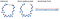As with Isomap, we can produce a graph (or network) of neighboring points.

Using ideas from Spectral Graph Theory, we can find a lower dimensional projection of the data while retaining the non-linear structure.

Again, we can approximate distances on the manifold using techniques in graph theory. We can do this by building a graph connecting each of our original data points to a set of neighboring points.

Laplacian Eigenmapping takes this graph and applies ideas from spectral graph theory to find a lower-dimensional embedding of the original data.

## Worked Example

OK, you’ve made it this far. Your reward is the chance to nerd out with our fourth and final dimensionality reduction algorithm. We’ll be exploring another non-linear technique. Like Isomap, it uses graph theory to approximate the underlying structure of the manifold. Check out the code.

Let’s start with similar spiral-shaped data to that we used before. But let’s make it even more tightly wound.

`set.seed(100)x <- y <- c(); a <- b <- 1for(i in 1:1000){    theta <- 0.02 * i    x <- append(x,(a+b*theta)*(cos(theta)+runif(1,-1,1))    y <- append(y,(a+b*theta)*(sin(theta)+runif(1,-1,1))}color <- rainbow(1200)[1:1000]spiral <- data.frame(x,y,color)plot(y~x, pch=20, col=color)`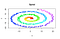The naive straight-line distance between A and B is much shorter than the distance from one end of the spiral to the other.

Linear techniques won’t stand a chance!

Again, we begin by constructing the adjacency matrix A of an n-nearest neighbors graph. n is a hyper-parameter we need to choose in advance.

Let’s try n = 10:

`n <- 10distance <- as.matrix(dist(spiral[,1:2]))A <- matrix(0,ncol=ncol(distance),nrow=nrow(distance))for(i in 1:nrow(A)){   neighbours <- as.integer(names(sort(distance[i,])[2:n+1]))   A[i,neighbours] <- 1}for(j in 1:nrow(A)){   for(k in 1:ncol(A)){      if(A[j,k] == 1){         out[k,j] <- 1      }   }}`

So far, so much like Isomap. We’ve added an extra few lines of logic to force the matrix to be symmetric. This will allow us to use ideas from spectral graph theory in the next step. We will define the Laplacian matrix of our graph.

We do this by building the degree matrix D:

`D <- diag(nrow(A))for(i in 1:nrow(D)){   D[i,i] = sum(A[,i])}`

This is a matrix the same size as A, where every element is equal to zero — except those on the diagonal, which equal the sum of the corresponding column of matrix A.

Next, we form the Laplacian matrix L with the simple subtraction:

`L = D - A`

The Laplacian matrix is another matrix representation of our graph particularly suited to linear algebra. It allows us to calculate a whole range of interesting properties.

To find our 1-D embedding of the original data, we need to find a vector x and eigenvalue λ.

This will solve the generalized eigenvalue problem:

`Lx = λDx`

Thankfully, you can put away the pencil and paper, because R provides a package to help us do this.

`install.packages('geigen'); require(geigen)eig <- geigen(L,D)eig\$values[1:10]`

We see that the `geigen() `function has returned the eigenvalue solutions from smallest to largest. Note how the first value is practically zero.

This is one of the properties of the Laplacian matrix — its number of zero eigenvalues tell us how many connected components we have in the graph. Had we used a lower value for n, we might have built a fragmented graph in say, three separate, disconnected parts — in which case, we’d have found three zero eigenvalues.

To find our low-dimensional embedding, we can take the eigenvectors associated with the lowest non-zero eigenvalues. Since we are projecting from 2-D into 1-D, we will only need one such eigenvector.

`embedding <- eig\$vectors[,2]plot(data.frame(embedding,1), col=spiral\$colors, pch=20)`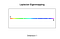We’ve even revealed the same ‘gaps’ as we saw in the original 2-D representation!

And there we have it — another non-linear data set successfully embedded in lower dimensions. Perfect!

We have implemented a simplified version of Laplacian Eigenmapping. We ignored choosing another hyper-parameter t, which would have had the effect of weighting our nearest-neighbors graph.

Take a look at the original paper for the full details and mathematical justification.

# Conclusion

There we are — a run through of four dimensionality reduction techniques that we can apply to linear and non-linear data. Don’t worry if you didn’t quite follow all the math (although congrats if you did!). Remember, we always need to strike a balance between theory and practice when it comes to data science.

These algorithms and several others are available in various packages of R, and in scikit-learn for Python.

Why, then, did we run through each one step-by-step? In my experience, rebuilding something from scratch is a great way to understand how it works.

Dimensionality reduction touches upon several branches of mathematics which are useful within data science and other disciplines. Putting these into practice is a great exercise for turning theory into application.

There are, of course, other techniques that we haven’t covered. But if you still have an appetite for more machine learning, then try out the links below:

Linear techniques:

Non-linear:

Thanks for reading! If you have any feedback or questions, please leave a response below!

Written by

## freeCodeCamp.org

#### This is no longer updated. Go to https://freecodecamp.org/news instead

Welcome to a place where words matter. On Medium, smart voices and original ideas take center stage - with no ads in sight. Watch
Follow all the topics you care about, and we’ll deliver the best stories for you to your homepage and inbox. Explore
Get unlimited access to the best stories on Medium — and support writers while you’re at it. Just \$5/month. Upgrade